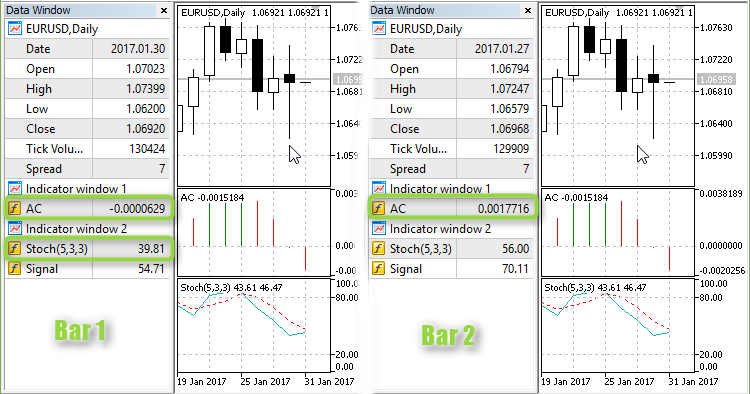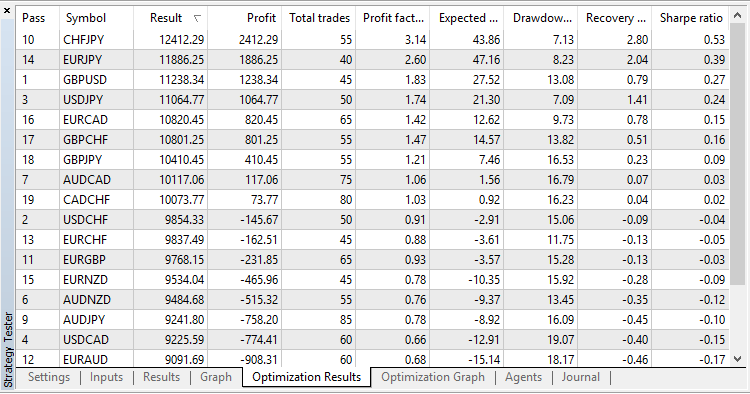# OzFx – expert for MetaTrader 5

• A+

Author of the idea:  Yuri, mq5 code author: barabashkakvn.

Description of generation of position open signals:

• Stoh_main_1 - the value of Stochastic Oscillator on bar #1.
• InpStochasticLevel - input for Stochastic level.
• AC_1 - the value of Accelerator Oscillator on bar #1.
• ACPrev_2 - the value of Accelerator Oscillator on bar #2.

BUY signal - when Stochastic Oscillator on bar #1 is greater than the Stochastic level input and Accelerator Oscillator on bar #1 is greater than Accelerator Oscillator on bar #2. In this case, Accelerator Oscillator on bar #1 should be above zero, and Accelerator Oscillator on #2 should be below zero:

```if(Stoh_main_1>InpStochasticLevel && AC_1>ACPrev_2 && AC_1>0 && ACPrev_2<0 && count_buys==0)
...
```

SELL signal - when Stochastic Oscillator on bar #1 is less than the Stochastic level input and Accelerator Oscillator on bar #1 is less than Accelerator Oscillator on bar #2. In this case, Accelerator Oscillator on bar #1 should be below zero, and Accelerator Oscillator on #2 should be above zero:

```if(Stoh_main_1<InpStochasticLevel && AC_1<ACPrev_2 && AC_1<0 && ACPrev_2>0 && count_sells==0)
...
```

An example of a SELL signal:When a signal is received, we open five positions with the same lot. The first position has Stop Loss and Take Profit equal to zero. All subsequent positions have equal Stop Loss and each one has an increasing Take Profit with the step of the input Take Profit (in pips).

Testing results on all symbols on PERIOD_D1: